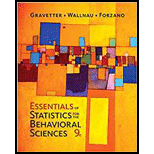# For a repeated-measures study with n – 12 scores in each treatment, a researcher constructs an 80% confidence interval to describe the mean difference between treatments. What value is at the center of the interval and what t values are used to construct the interval? a. The sample mean difference is at the center and t = ± 1.363. b. The sample mean difference is at the center and t = ± 1.316. c. Zero is at the center and t = ± 1.363. d. Zero is at the center and t = ± 1.316.### Essentials of Statistics for The B...

9th Edition
Frederick J Gravetter + 2 others
Publisher: Cengage Learning
ISBN: 9781337098120

#### Solutions

Chapter
Section### Essentials of Statistics for The B...

9th Edition
Frederick J Gravetter + 2 others
Publisher: Cengage Learning
ISBN: 9781337098120
Chapter 11.4, Problem 2LC
Textbook Problem
1 views

## For a repeated-measures study with n – 12 scores in each treatment, a researcher constructs an 80% confidence interval to describe the mean difference between treatments. What value is at the center of the interval and what t values are used to construct the interval?a. The sample mean difference is at the center and t = ± 1.363.b. The sample mean difference is at the center and t = ± 1.316.c. Zero is at the center and t = ± 1.363.d. Zero is at the center and t = ± 1.316.

To determine

To Find: The value at the centre of the interval.

The t values used to construct the interval.

### Explanation of Solution

Given info:

Numbers of scores in each treatment are n=12 .

80% confidence interval is given.

Calculation:

Let n be the numbers of participants in the treatment. Then,

n=12

80% confidence interval is given which means two tailed level of significance is 20%.

Let μD , sMD , t, and MD be the population mean difference, standard error of sample mean difference, critical values and sample mean difference of given two tailed test.

Then, 80% confidence limits are given by the formulae:

μD=MD±t×sMDWhere t is the upper 20% critical values with  (n1)degree of freedom.

Therefore, sample mean difference is at the centre of the confidence interval

### Still sussing out bartleby?

Check out a sample textbook solution.

See a sample solution

#### The Solution to Your Study Problems

Bartleby provides explanations to thousands of textbook problems written by our experts, many with advanced degrees!

Get Started

Find more solutions based on key concepts
In Exercises 7-12, solve for y in terms of x. x=2y14

Calculus: An Applied Approach (MindTap Course List)

Prove the third law of exponents for ex [see (11)].

Single Variable Calculus: Early Transcendentals, Volume I

In Exercises 7984, determine whether the statement is true or false for all real numbers a and b. 83. |a + b| =...

Applied Calculus for the Managerial, Life, and Social Sciences: A Brief Approach

Find each product. 5x(8x2x+5)

Elementary Technical Mathematics

Given that for all x, a power series for is:

Study Guide for Stewart's Multivariable Calculus, 8th

True or False: If a number p is in the interval of convergence of then so is the number .

Study Guide for Stewart's Single Variable Calculus: Early Transcendentals, 8th